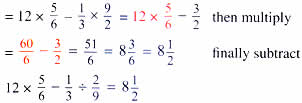Friday , July 10 2020# NCERT 5th Class (CBSE) Mathematics: Order Of Operations

(c) 12×5/6-1/3÷2/9

= 12×5/6-1/3÷2/9         first divide#### ‘Of’ → Division → Multiplication → Addition → Subtraction

48÷2/3 of 72×5

= 48÷2/3 of 72×5 first perform the ‘of’ operation

= 48÷2/3×72×5

= 48÷48×5 = 1×5          then divide

= 1×5 = 5             finally multiply

48÷2/3 of 72×5 = 5

#### Brackets → ‘of’ → Division → Multiplication → Addition →  Subtraction

Brackets group operations and must be simplified first.

Look at the following examples:

(a) 7+2 × ( 8 – 2 )

= 7 + 2 × ( 8 -2 )          first do the operation within the brackets

= 7 + 2 × 6                    next, multiply

= 7 + 12 = 19                finally, add

7 + 2 × (8 – 2) = 19

## 10th Class CBSE Mathematics 2018-19

10th Class CBSE Mathematics 2018-19 Time: 3 hours M.M.: 80 Class: 10th Subject: Mathematics Date: 07/03/2019 …